Consider allowing JavaScript. Otherwise, you have to be proficient in readingsince formulas will not be rendered. Furthermore, the table of contents in the left column for navigation will not be available and code-folding not supported. Sorry for the inconvenience.

Examples in this article were generated with4.2.1 by the package PowerTOST.1

• Click to show / hide R code.

# Introduction

Have you ever wondered where all these nice numbers come from?

$$\small{\alpha\;0.05}$$, bioequivalence limits $$\small{80.00-125.00\%}$$, switching to SABE at $$\small{CV_\textrm{wR}\;30\%}$$, $$\small{k\;0.760}$$, upper cap of scaling at $$\small{CV_\textrm{wR}\;50\%}$$, $$\small{s_\textrm{wR}\;0.294}$$, you name it.

The list is endless and as interesting as a telephone directory.

# What the Heck?

## α 0.05

Why this number? OK, at least this one is easy to explain: Mr. Fisher considered it convenient.

The value for which p = 0.05, or 1 in 20, is 1.96 or nearly 2; it is convenient to take this point as a limit in judging whether a deviation is to be considered significant or not.
Ronald A. Fisher (1925)2

For nearly a century this number is the holy grail of frequentists. Members of the other chuch, the Bayesians, hold other believes.Fig. 1 XXVIIth International Biometric Conference. Florence. July 6–11, 2014.

## 90% Confidence Interval

Did you ever wonder why (based on Mr Fisher’s $$\small{\alpha=0.05}$$) we use a $$\small{100\,(1-2\,\alpha)=90\%}$$ confidence interval in bioequivalence and not the $$\small{95\%}$$ CI like in clinical Phase III?

In Phase III we want to demonstrate superiority (for nitpickers: non-inferiority) of verum to placebo. Once a drug is approved, we can be ≥95% sure that it is safe and efficacious (though there is a ≤5% chance that even ‘blockbuster drug’ does not perform better than snake-oil).

In the early days of BE a $$\small{95\%}$$ CI was applied indeed. Below a scan of my conference proceedings.3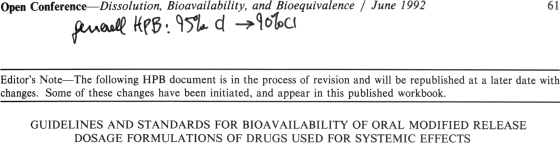Fig. 2 Note my handwritten comment (“generell” is German for generally).
The guideline contained five text passages stating
»The 95% confidence interval…«

So why did we change to the $$\small{90\%}$$ CI?

In a particular patient the bioavailability can be
either too low $$\small{(p(\textrm{BA}<80\%)>5\%)}$$
or too high $$(\small{p(\textrm{BA}>125\%)>5\%})$$

but evidently not at the same time.
The $$\small{90\%}$$ CI controls the risk for the population of patients. Therefore, if a study passes, the risk for patients does still not exceed $$\small{5\%}$$.4 5 6

sd   <- 0.15
n    <- 501
ylim <- c(0, dnorm(x = 0, sd = sd))
dev.new(width = 4.5, height = 2.25, record = TRUE)
par(mar = c(4, 1, 0, 0), cex.axis = 0.9)
# TOST (left)
plot(log(c(0.5, 2)), c(0, 0), type = "n", xlim = log(c(0.5, 2)), axes = FALSE,
ylim = ylim, xlab = expression(mu[T]/mu[R]*" (%)"), ylab = "")
axis(1, at = c(log(0.5), log(1/1.5), log(0.8), 0, log(1.25), log(1.5), log(2)),
labels = sprintf("%.0f", 100*c(0.5, 1/1.5, 0.8, 1, 1.25, 1.5, 2)))
mtext(side = 2, text = expression(italic(p)*" (%)"), line = -0.1)
x <- seq(log(0.8), log(2), length.out = n)
polygon(x = c(x, rev(x)), y = c(rep(0, n), rev(dnorm(x = x, sd = sd))),
col = "#90EE90", border = "#90EE90")
text(0, ylim/2, adj = 0.5, labels = 95, cex = 0.9)
x <- seq(log(0.5), log(0.8), length.out = n)
polygon(x = c(x, rev(x)), y = c(rep(0, n), rev(dnorm(x = x, sd = sd))),
col = "#FA8072", border = "#FA8072")
text(log(0.8), dnorm(x = log(1.25), sd = sd)*0.2, pos = 2, offset = 0.5,
labels = 5, cex = 0.9)
# TOST (right)
plot(log(c(0.5, 2)), c(0, 0), type = "n", xlim = log(c(0.5, 2)), axes = FALSE,
ylim = ylim, xlab = expression(mu[T]/mu[R]*" (%)"), ylab = "")
axis(1, at = c(log(0.5), log(1/1.5), log(0.8), 0, log(1.25), log(1.5), log(2)),
labels = sprintf("%.0f", 100*c(0.5, 1/1.5, 0.8, 1, 1.25, 1.5, 2)))
mtext(side = 2, text = expression(italic(p)*" (%)"), line = -0.1)
x <- seq(log(0.5), log(1.25), length.out = n)
polygon(x = c(x, rev(x)), y = c(rep(0, n), rev(dnorm(x = x, sd = sd))),
col = "#90EE90", border = "#90EE90")
text(0, ylim/2, adj = 0.5, labels = 95, cex = 0.9)
x <- seq(log(1.25), log(2), length.out = n)
polygon(x = c(x, rev(x)), y = c(rep(0, n), rev(dnorm(x = x, sd = sd))),
col = "#FA8072", border = "#FA8072")
text(log(1.25), dnorm(x = log(1.25), sd = sd)*0.2, pos = 4, offset = 0.5,
labels = 5, cex = 0.9)
# 90% CI inclusion
plot(log(c(0.5, 2)), c(0, 0), type = "n", xlim = log(c(0.5, 2)), axes = FALSE,
ylim = ylim, xlab = expression(mu[T]/mu[R]*" (%)"), ylab = "")
axis(1, at = c(log(0.5), log(1/1.5), log(0.8), 0, log(1.25), log(1.5), log(2)),
labels = sprintf("%.0f", 100*c(0.5, 1/1.5, 0.8, 1, 1.25, 1.5, 2)))
mtext(side = 2, text = expression(italic(p)*" (%)"), line = -0.1)
x <- seq(log(0.8), log(1.25), length.out = n)
polygon(x = c(x, rev(x)), y = c(rep(0, n), rev(dnorm(x = x, sd = sd))),
col = "#90EE90", border = "#90EE90")
text(0, ylim/2, adj = 0.5, labels = 90, cex = 0.9)
x <- seq(log(0.5), log(0.8), length.out = n)
polygon(x = c(x, rev(x)), y = c(rep(0, n), rev(dnorm(x = x, sd = sd))),
col = "#FA8072", border = "#FA8072")
text(log(0.8), dnorm(x = log(1.25), sd = sd)*0.2, pos = 2, offset = 0.5,
labels = 5, cex = 0.9)
x <- seq(log(1.25), log(2), length.out = n)
polygon(x = c(x, rev(x)), y = c(rep(0, n), rev(dnorm(x = x, sd = sd))),
col = "#FA8072", border = "#FA8072")
text(log(1.25), dnorm(x = log(1.25), sd = sd)*0.2, pos = 4, offset = 0.5,
labels = 5, cex = 0.9)
par(op)Fig. 3 90% confidence interval unveiled.

If we would have kept the $$\small{95\%}$$ CI, the patient’s risk would be only $$\small{2.5\%}$$. Fine in principle but we are only $$\small{95\%}$$ confident that the reference product works at all (see above). Hence, keeping the $$\small{95\%}$$ CI would have been double standards.

## 80 – 125%

In the early days of bioequivalence, the analysis was performed on raw (untransformed) data, assuming an additive model. Based on a clinically not relevant difference $$\small{\Delta=20\%}$$7 one gets: $\left\{\theta_1,\theta_2\right\}=\left\{100\,(1-\Delta),100\,(1+\Delta)\right\}=80-120\%\tag{1}$ One should not forget that bioequivalence was never a scientific theory in the Popperian sense but an ad hoc solution to a pressing problem in the 1970s.8 9 The commonly assumed clinically not relevant difference $$\small{\Delta=20\%}$$ is arbitrary (as any other). However, we have decades of empiric evidence that the concept is sufficient in practice. Apart from occasional anecdotal reports (mainly dealing with narrow therapeutic index drugs), no problems are evident switching between the originator and generics (and vice versa) in terms of lacking efficacy and safety problems.

Some argued that concentrations and derived pharmacokinetic metrics like the Area Under the Curve (AUC) do not follow a normal distribution – which is a prerequiste of the ANOVA. Makes sense.
If one works with the arithmetic mean and its variance, it implies that there is a certain probability of negative values because the domain of $$\small{\mathcal{N}(\mu;\sigma^2)}$$ is $$\small{[-\infty<x+\infty]}$$ for $$\small{x\in \mathbb{R}}$$.

A captivating example of the FDA.10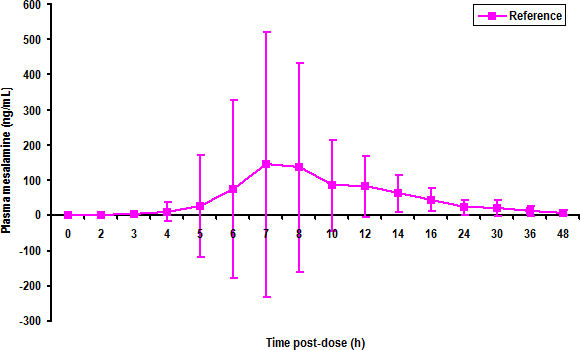Fig. 4 $$\small{\overline{x}_\textrm{ar}\pm \textrm{s}\;(n=238)}$$.
$$\small{\textrm{t}=0,2,3,4,5,6,7,8,10,12,14,16,24,30,36,48\;\textrm{h}}$$
$$\small{\Delta_\textrm{t}=2,1,1,1,1,1,1,2,2,2,2,8,6,6,12\;\textrm{h}}$$

Splendid, a line chart instead of a scatter plot.
Oh dear, someone clicked the first (in Excel-parlance a line plot instead of an XY plot)! Therefore, one hour intervals in the early part of the profile appear as wide as the twelve at the end. Yep, in a line chart the x-values are ordered and the axis is equally spaced. That gives the false impression that the drug follows zero-order elimination. Nope, it wasn’t C2H5OH…Any (descriptive) statistic implies an underlying distribution $$\small{(\overline{x}_\textrm{ar},\,\textrm{s}\;\mapsto\textrm{normal}})$$.
Did these folks really believe that at seven hours there’s a ≈16% probability that concentrations are ≤–232 ng/mL

Which cult of Pastafarianism do they belong to? The one holding that negative mass exist or the other believing in negative lengths?

Exploring large data sets we see that the distribution is skewed to the right. The lognormal distribution does not only pull the right tail in but also with its domain of $$\small{]0<x+\infty]}$$ for $$\small{x\in \mathbb{R}^{+}}$$ avoids the ‘problem’ of negative concentrations. The location parameter is the geometric mean.Fig. 5 $$\small{\overline{x}_\textrm{geom}\pm \textrm{s}_\textrm{geom}}$$.

This plot reflects the extreme variability of the drug much better and shows that high concentrations are more likely than low ones. Of course, we see also that elimination is first-order (exponentially decreasing).

After a dose we know only one thing for sure: The concentration is not zero.
Harold Boxenbaum (1990)11

His statement ended in shouting matches. Don’t know why.12

<nitpick>
The distribution of data per se is not important. Only the model’s residual errors – as estimates of the true errors $$\epsilon$$ – have to be normally distributed. However, exploring large data sets again, we see that when performing the analysis on untransformed data that they aren’t.

</nitpick>

Another argument is based on the fundamental equation of pharmacokinetics, namely $AUC=\frac{f\cdot D}{CL},\tag{2}$ where $$\small{f}$$ is the fraction absorbed, $$\small{D}$$ the dose, and $$\small{CL}$$ the clearance.

If we want to compare the bioavailabilities of two drugs ($$\small{f_\textrm{T},f_\textrm{R}}$$) we arrive at $\frac{f_\textrm{T}}{f_\textrm{R}}=\frac{AUC_\textrm{T}\cdot CL}{D_\textrm{T}}\Big{/}\frac{AUC_\textrm{R}\cdot CL}{D_\textrm{R}}.\tag{3}$ By assuming13 identical doses ($$\small{D_\textrm{T}\equiv D_\textrm{R}}$$) and no inter-occasion variability of clearances ($$\small{CL=\textrm{const}}$$) we can cancel both out $\require{cancel}\frac{f_\textrm{T}}{f_\textrm{R}}=\frac{AUC_\textrm{T}\cdot \cancel{CL}}{\cancel{D_\textrm{T}}}\Big{/}\frac{AUC_\textrm{R}\cdot \cancel{CL}}{\cancel{D_\textrm{R}}}\tag{4}$ to get what we want: $\frac{f_\textrm{T}}{f_\textrm{R}}=\frac{AUC_\textrm{T}}{AUC_\textrm{R}}.\tag{5}$ Hey, that’s a ratio and hence, we have to use a multiplicative model.

But we need differences in the ANOVA (which is an additive model)… Nothing easier than that. $$\small{(2)}$$ can be rewritten to $\log_{e}AUC=\log_{e}f\,+\log_{e}D\,-\log_{e}CL.\tag{6}$ After the same substitutions and cancelations as in $$\small{(3)}$$ and $$\small{(4)}$$, perform the analysis $\log_{e}PE=\log_{e}f_T\,-\log_{e}f_T=\log_{e}AUC_\textrm{T}\,-\log_{e}AUC_\textrm{R},\tag{7}$ and use $$\small{\exp(\log_{e}PE)}$$ to get with $$\small{PE}$$ a number clinicians can comprehend.14

At the first BioInternational conference15 there was a poll among the participants about the transformation. Outcome: ⅓ never, ⅓ always, ⅓ case by case (i.e., perform both analyses and report the one with narrower confidence interval ‘because it fits the data better’). Let’s be silent about the last camp.16

Wait a minute! The original acceptance range $$\small{(1)}$$ was symmetrical around $$\small{100\%}$$. In $$\small{\log_{e}}$$-scale it should be symmetrical around $$\small{0}$$ (because $$\small{\log_{e}1=0}$$).
What happens to our $$\small{\Delta}$$ which should still be $$\small{20\%}$$? Due to the positive skewness of the lognormal distribution a [heated debate] lively discussion started after early publications proposing $$\small{80-125\%}$$.17 18 Keeping $$\small{80-120\%}$$ would be flawed because the maximum power should be obtained at $$\small{\mu_\textrm{T}/\mu_\textrm{R}=1}$$ for $\exp\left((\log_{e}\theta_1+\log_{e}\theta_2)/2\right),\tag{8}$ which works only if $$\small{\theta_2=\theta_1^{-1}}$$ or $$\small{\theta_1=\theta_2^{-1}}$$. Keeping the original limits, maximum power would be obtained at $$\small{\mu_\textrm{T}/\mu_\textrm{R}=\exp((\log_{e}0.8+\log_{e}1.2)/2)\approx0.979796}$$.Fig. 6 Power curve for a 2×2×2 design, $$\small{n=28}$$.
Note that the x-axis is in log-scale.

There were three parties (all agreed that the acceptance range should be symmetrical in $$\small{\log_{e}}$$-scale and consequently asymmetrical when back-transformed).
These were their suggestions:

$\left\{\theta_1,\theta_2\right\}=81.98-121.98\%\tag{9}$ $\left\{\theta_1,\theta_2\right\}=\left\{100/(1+\Delta),100\,(1+\Delta)\right\}=8\dot{3}.33-120\%\tag{10}$ $\left\{\theta_1,\theta_2\right\}=\left\{100\,(1-\Delta),100/(1-\Delta)\right\}=80-125\%\tag{11}$

• The argument of the first party was essentially:
The width of the acceptance range was 40% and we have empiric evidence that the concept of bioequivalence ‘worked’ – let’s keep it.

• The second party argued:
Since that’s a new method we don’t want to face safety issues with a higher limit. Furthermore, a more restrictive lower limit prevents issues with insufficient efficacy.

• The third party argued:
80% as the lower limit served us well in the past. Hence, 125% is the way to go because it is simply the reciprocal of the lower limit and the coverage probability in the log-domain is the same like the one we had.
Furthermore, these are nice numbers.

We all know which party prevailed. Can you imagine why?

previous section ↩︎

## To Round or not to Round

How I love rounding of the confidence interval according to the guidelines!
All jurisdictions require the result in percent rounded to two decimal figures, whereas Health Canada to only one.

If we do that19 it will increase the patient’s risk. Why? Simple: If we would use the exact result, a study with a lower confidence limit of $$\small{79.995\%}$$ would fail as one would with an upper confidence limit of say, $$\small{125.005\%}$$. Both would pass after rounding (for details see the Interlude below).

library(PowerTOST) # attach it to run the examples
# Cave: long runtime
# Exact rounding according to IEEE 754
theta0 <- 0.95 # assumed T/R-ratio
CV0    <- 0.25 # assumed CV
target <- 0.80 # target power
design <- "2x2x2"
nsims  <- 1e5L # number of simulations
set.seed(123456)
n      <- sampleN.TOST(CV = CV0, theta0 = theta0, targetpower = target,
design = design, print = FALSE)[["Sample size"]]
CV     <- mse2CV(CV2mse(CV0) * rchisq(nsims, df = n - 2) / (n - 2))
pe     <- exp(rnorm(nsims, mean = log(theta0),
sd = sqrt(0.5 / n) * sqrt(CV)))
comp   <- data.frame(CV = CV, PE = 100 * pe, lower = NA_real_,
upper = NA_real_, exact = FALSE, round2 = FALSE,
round1 = FALSE, round0 = FALSE)
for (i in 1:nsims) {
comp[i, 3:4] <- 100*CI.BE(CV = CV[i], pe = pe[i], n = n)
if (comp$lower[i] >= 80 & comp$upper[i] <= 125) comp$exact[i] <- TRUE if (round(comp$lower[i], 2) >= 80 & round(comp$upper[i], 2) <= 125) comp$round2[i] <- TRUE
if (round(comp$lower[i], 1) >= 80 & round(comp$upper[i], 1) <= 125)
comp$round1[i] <- TRUE if (round(comp$lower[i], 0) >= 80 & round(comp$upper[i], 0) <= 125) comp$round0[i] <- TRUE
}
cat("Design      :", design,
"\nCV          :", CV0,
"\ntheta0      :", theta0,
"\ntarget power:", target,
"\nn           :", n,
"\nPercentage of", formatC(nsims, format = "d", big.mark = ","),
"simulated studies passing\nthe acceptance limits with the 90% CI:",
"\n  full precision:",
sprintf("%.3f%% (scientific)", 100 * sum(comp$exact) / nsims), "\n 80.00 \u2013 125.00:", sprintf("%.3f%% (guidelines)", 100 * sum(comp$round2) / nsims),
"\n  80.0  \u2013 125.0 :",
100 * sum(comp$round1) / nsims), "\n 80 \u2013 125 :", sprintf("%.3f%% (only for comparison)", 100 * sum(comp$round0) / nsims), "\n")
# Design      : 2x2x2
# CV          : 0.25
# theta0      : 0.95
# target power: 0.8
# n           : 28
# Percentage of 100,000 simulated studies passing
# the acceptance limits with the 90% CI:
#   full precision: 80.341% (scientific)
#   80.00 – 125.00: 80.375% (guidelines)
#   80.0  – 125.0 : 80.605% (Health Canada)
#   80    – 125   : 82.812% (only for comparison)

Do you see a pattern? Am I picky?
In all textbooks and publications the confidence interval inclusion approach is given as $\begin{matrix}\tag{12} \theta_1=1-\Delta,\theta_2=\left(1-\Delta\right)^{-1}\\ H_0:\;\frac{\mu_\textrm{T}}{\mu_\textrm{R}}\not\subset\left\{\theta_1,\,\theta_2\right\}\;vs\;H_1:\;\theta_1<\frac{\mu_\textrm{T}}{\mu_\textrm{R}}<\theta_2, \end{matrix}$ where $$\small{H_0}$$ is the null hypothesis of inequivalence and $$\small{H_1}$$ the alternative hypothesis. $$\small{\theta_1}$$ and $$\small{\theta_2}$$ are the lower and upper limits of the acceptance range, and $$\small{\mu_\textrm{T}}$$ and $$\small{\mu_\textrm{R}}$$ the geometric least squares means of $$\small{\textrm{T}}$$ and $$\small{\textrm{R}}$$, respectively.
Alternatively by the TOST procedure:20 $\begin{matrix}\tag{13} H_{01}:\frac{\mu_\textrm{T}}{\mu_\textrm{R}}\leq \theta_1 & vs & H_{11}:\frac{\mu_\textrm{T}}{\mu_\textrm{R}}>\theta_1\\ H_{02}:\frac{\mu_\textrm{T}}{\mu_\textrm{R}}\geq \theta_2 & vs & H_{12}:\frac{\mu_\textrm{T}}{\mu_\textrm{R}}<\theta_2 \end{matrix}$ Do you see anything about rounding here? I don’t. If you can point me to a publication supporting this regulatory ‘invention’, please let me know.

In older releases of Phoenix WinNonlin there was a verbatim statement based on the confidence interval in full precision like
Average bioequivalence shown for confidence=0.90 and percent=0.20
or
Failed to show average bioequivalence for confidence=0.90 and percent=0.20
After rounding according to the guidelines that may not be ‘correct’ any more. I mused about writing a one-sentence SOP:
»Delete the verbatim bioequivalence assessment from the output
because it might contradict rounded results and provoke questions.
«

For good reasons Certara removed the text from the output in later releases.

Interlude

If you insist in rounding (I hope, you don’t), do it at least correctly, i.e., according to the IEEE Standard for Floating-Point Arithmetic. That’s also called ‘Round to Even’, where zero is considered an even number.
Let’s call the digit at the desired decimal place x and the digit after the desired decimal place y. Rules:

1. If y is 0, 1, 2, 3, 4 →
round x down.
2. If y is 5 (follow by other digits), 6, 7, 8, 9 →
round x up.
3. If y is 5 →
round x in such a way that it becomes an even number.
Examples of rounding numbers close to the upper BE-limit to two decimal figures according to the guidelines:
125.004 125.00 acc. to 1.
125.0051125.01 acc. to 2.
125.005 125.00 acc. to 3.

That’s not necessarily the default in some software and in others it is not implemented at all. Instead, rounding ‘Away from Zero’ (commercial rounding, as we are taught at school) is used.

Let’s see what happens to 125.005 (according to rule 3, 125.00 is correct).

Software Function Result Note
Excel ROUND(125.005, 2) 125.01 All versions
MROUND(125.005, 0.01) 125.01 All versions
Other spreadsheets ROUND(125.005, 2) 125.01 All versions
Phoenix Win­Nonlin round(125.005, 2) 125.01 All versions
JavaScript Math.round(125.005 * 100) / 100 125.01 All versions
GNU Octave round(125.005 * 100) / 100 125.01 All versions
PHP < 5.3.0 round(125.005, 2) 125.01 Only implemented
PHP ≥ 5.3.0 round(125.005, 2) 125 New default
round(125.005, 2, PHP_ROUND_HALF_EVEN) 125 Preferrable for clarity
round(125.005, 2, PHP_ROUND_HALF_UP) 125.01 Back-compatability
SAS ROUND(125.005, 0.01) 125.01
ROUNDE(125.005, 0.01) 125
C round(125.005 * 100) / 100 125.01
lround(125.005 * 100) / 100 125.01
printf("%.2f", 125.005) 125.00
C++ round(125.005 * 100) / 100 125.01
lround(125.005 * 100) / 100 125
Java Math.round(125.005 * 100) / 100 125
Perl printf("%.2f", 125.005) 125.00
SQLite SELECT round(125.005, 2) 125.0
WolframAlpha round(125.005, 0.01) 125
Maxima round(125.005 * 100) / 100 125
R round(125.005, 2) 125
julia round(125.005; digits = 2, base = 10) 125.0
Python round(125.005, 2) 125.0
∗ Compiler and variable declarations not shown

For correct rounding in spreadsheets use ROUND(125.005, 4-(1+INT(LOG10(ABS(125.005))))). In other software (Phoenix Win­Nonlin, JavaScript, GNU Octave, C) you have to roll out your own functions as well.

If you want to get – for comparison purposes – commercial rounding in R:

c.round <- function(x, y) sign(x) * trunc(abs(x) * 10^y + 0.5) / 10^y
c.round(125.005, 2)
#  125.01

In IEEE 754-compliant software (Java, Perl, SQLite, WolframAlpha, Maxima, julia, Python) you have also to roll out similar functions for commercial rounding.

After rounding a ‘worst case’ confidence interval the width of the acceptance range will appear as the expected 45% but actually it is wider. The clinically relevant difference $$\small{\Delta=20\%}$$ is not kept (the ‘implied’ limits are the maximum possible ones for rounding, i.e., 79.995 – 125.005%).

AR <- AR.new <- setNames(c(80, 125), c("lower", "upper"))
# True acceptance limits due to rounding
AR.new[1:2]  <- c(79.995, 125.005)
rd       <- round(AR.new, 2)
BE.CI    <- BE.rd <- "fail"
if (AR.new >= AR & AR.new <= AR) BE.CI <- "pass"
if (rd >= AR & rd <= AR) BE.rd <- "pass"
# Examples
CV       <- seq(0.15, 0.3, 0.025)
res1     <- res2 <- data.frame(CV = CV, n = NA_integer_,
lower = NA_real_, upper = NA_real_)
# Use the defaults: design = 2x2x2, theta0 = 0.95, targetpower = 0.8
for (i in seq_along(CV)) {
res1$n[i] <- sampleN.TOST(CV = CV[i], print = FALSE)[["Sample size"]] res1$lower[i] <- power.TOST(CV = CV[i], n = res1$n[i], theta0 = 0.80, theta1 = AR.new[["lower"]] / 100, theta2 = AR.new[["upper"]] / 100) res1$upper[i] <- power.TOST(CV = CV[i], n = res1$n[i], theta0 = 1.25, theta1 = AR.new[["lower"]] / 100, theta2 = AR.new[["upper"]] / 100) res2$n[i]     <- sampleN.TOST(CV = CV[i],
theta1 = AR.new[["lower"]] / 100,
theta2 = AR.new[["upper"]] / 100,
print = FALSE)[["Sample size"]]
res2$lower[i] <- power.TOST(CV = CV[i], n = res2$n[i],
theta0 = AR.new[["lower"]] / 100,
theta1 = AR.new[["lower"]] / 100,
theta2 = AR.new[["upper"]] / 100)
res2$upper[i] <- power.TOST(CV = CV[i], n = res2$n[i],
theta0 = AR.new[["lower"]] / 100,
theta1 = AR.new[["lower"]] / 100,
theta2 = AR.new[["upper"]] / 100)
}
cat("Acceptance range:",
paste(sprintf("%.2f%%", AR), collapse = " , "),
sprintf(" (midpoint: %9.5f%%)", sqrt(prod(AR))),
"\nTrue AR         :",
paste(sprintf("%.3f%%", AR.new), collapse = ", "),
sprintf("(midpoint: %9.5f%%)", sqrt(prod(AR.new))),
"\nRounded AR      :",
paste(sprintf("%5.2f%%", rd), collapse = " , "),
"\n\nExpected width of acceptance range:",
sprintf("%.2f%%", diff(AR)),
sprintf("(Delta %.3f%%)", 100 - AR),
"\nTrue width of acceptance range    :",
sprintf("%.2f%%", diff(AR.new)),
sprintf("(Delta %.4f to %.3f%%)",
100 * (1 - 100 / AR.new), 100 - AR.new),
"\n\nBE based on extreme CI     :", BE.CI,
"\nBE based rounded extreme CI:", BE.rd)
cat("\n\nType I Errors at \u2013 exactly \u2013 80 and 125% (Delta 20%)\n")
print(round(res1, 7), row.names = FALSE)
cat("Type I Errors at the \u2018implied\u2019 limits 79.995 and 125.005%\n")
print(round(res2, 7), row.names = FALSE)
# Acceptance range: 80.00% , 125.00%  (midpoint: 100.00000%)
# True AR         : 79.995%, 125.005% (midpoint:  99.99887%)
# Rounded AR      : 80.00% , 125.00%
#
# Expected width of acceptance range: 45.00% (Delta 20.000%)
# True width of acceptance range    : 45.01% (Delta 20.0032 to 20.005%)
#
# BE based on extreme CI     : fail
# BE based rounded extreme CI: pass
#
# Type I Errors at – exactly – 80 and 125% (Delta 20%)
#     CV  n     lower     upper
#  0.150 12 0.0500989 0.0500632
#  0.175 16 0.0501000 0.0500640
#  0.200 20 0.0500991 0.0500634
#  0.225 24 0.0500973 0.0500622
#  0.250 28 0.0500951 0.0500607
#  0.275 34 0.0500962 0.0500615
#  0.300 40 0.0500963 0.0500615
# Type I Errors at the ‘implied’ limits 79.995 and 125.005%
#     CV  n     lower     upper
#  0.150 12 0.0499998 0.0499998
#  0.175 16 0.0499999 0.0499999
#  0.200 20 0.0499999 0.0499999
#  0.225 24 0.0499998 0.0499998
#  0.250 28 0.0499996 0.0499996
#  0.275 34 0.0499997 0.0499997
#  0.300 40 0.0499998 0.0499998

Therefore, with rounding the probability of passing BE increases and will lead to an inflated Type I Error. Note that we see different Type I Errors at $$\small{\left\{\theta_1,\,\theta_2\right\}}$$ because the ‘implied’ limits are not symmetrical in log-scale.

Hence, the assessment for BE should always be performed with the CI in full numeric precision. Only then the Type I Error is strictly controlled.

## 90.00 – 111.11%

These limits are stated in most guideline when dealing with Narrow Therapeutic Index Drugs (NTIDs). Should we take the upper limit literally and not based on $$\small{\Delta=10\%}$$? $\left\{\theta_1,\theta_2\right\}=\left\{100\,(1-\Delta),100/(1-\Delta)\right\}=90\%,\dot{1}11.11\%\tag{14}$ If yes, we would have to apply double rounding (of the upper limit and the CI), which should be avoided according to IEEE 754-2008.

## 90.0 – 112.0%

Health Canada requires for ‘critical dose drugs’ (i.e., NTIDs) that the confidence interval of AUC lies within $$\small{90.0-112.0\%}$$.22 I always thought that $$\small{100/0.900=\dot{1}11.11\%}$$.

On the long run products will be approved with $$\small{\sqrt{90.0\times112.0}\approx 100.4\%}$$. When asked, the reply was:
»These numbers are more easy to remember.«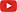»You cannot be serious!«

## 75.00 – 133.33%

A similar story, this time dealing with widening the limits for Highly Variable Drugs / Drug Products.23 24 25 Based on $$\small{\Delta=25\%}$$: $\left\{\theta_1,\theta_2\right\}=\left\{100\,(1-\Delta),100/(1-\Delta)\right\}=75\%,1\dot{3}3.33\%\tag{15}$ Double rounding again?

## k 0.760

All jurisdictions accepting Average Bioequivalence with Expanding Limits (ABEL) for HVD(P)s give the regulatory constant $$\small{k}$$ in the expansion formula $\left\{\theta_1,\theta_2\right\}=100\exp(\mp k\,\cdot s_\textrm{wR})\tag{16}$ with $$\small{0.760}$$. Where does it come from?

Based on the switching $$\small{CV_0=30\%}$$ we get $k=\log_{e}1.25 \Big{/}\sqrt{\log_{e}(CV_0^{2}+1)}\approx 0.7601283\ldots\tag{17}$ Just another ‘nice’ number. If we would back-transform $$\small{k=0.760}$$, the switching $$\small{CV_0}$$ would be $$\small{30.00529\%}$$ and not the claimed $$\small{30\%}$$.26

## Upper cap 57.4%

Health Canada gives the upper cap of scaling in ABEL with $$\small{CV_\textrm{wR}\;57.4\%}$$ but state also that the expansion is limited to $$\small{66.7-150.0\%}$$.27 ‘Nice’ numbers as usual but there’s a contradiction.

With $$\small{CV_\textrm{wR}\;57.4\%}$$ we get \eqalign{\tag{18} s_\textrm{wR}&=\sqrt{\log_{e}(0.574^2+1)}&\approx 0.5336524\ldots\\ \left\{\theta_{\textrm{s}_1},\theta_{\textrm{s}_2}\right\}&=100\exp(\mp0.760\cdot s_\textrm{wR})&\approx 66.65929\%,150.0166\% \eqalign} In order to obtain $$\small{66.7-150.0\%}$$, in the power and sample size functions of PowerTOST we decided to use a cap of $$\small{0.57382}$$ instead.

hc <- reg_const(regulator = "HC")
print(hc)
round(100*scABEL(CV = hc\$CVcap, regulator = "HC"), 1)
# HC regulatory settings
# - CVswitch            = 0.3
# - cap on scABEL if CVw(R) > 0.57382
# - regulatory constant = 0.76
# - pe constraint applied
# lower upper
#  66.7 150.0

It’s guesswork. In coding the package PowerTOST we assumed that $$\small{66.7-150.0\%}$$ is more ‘important’ than a cap at exactly $$\small{CV_\textrm{wR}=57.4\%}$$.

## s0 0.294

A similar goody in the FDA’s Reference-Scaled Average Bioequivalence (RSABE).28 29 Again we have the switching $$\small{CV_0=30\%}$$. But then $s_0=\sqrt{\log_{e}(CV_0^{2}+1)}\approx 0.2935604\ldots\tag{19}$ I thought that there is a consensus about the classification of HVD(P)s, i.e., $$\small{CV_0\;30\%}$$. Not for the FDA, giving the regulatory constant with $$\small{0.294}$$, which translates to $$\small{30.04689\%}$$.

## σw0 0.25

There is a consensus about $$\small{CV_\textrm{wR}=30\%}$$ classifying drugs as highly variable30 but ones with $$\small{CV_\textrm{wR}=25\%}$$ were considered ‘problematic’.31

The FDA prefers the ‘nicer’ $$\small{\sigma_\textrm{w0}\;0.25}$$, which translates to $$\small{25.39576\%}$$.

# Utopia

Instead of ‘nice’ numbers guidelines should state the formulas, which are not more than elementary maths any eight grader could master. It’s not rocket science. We are not retarded.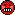All we need are exact numbers of $$\small{\Delta}$$ in ABE and $$\small{CV_0/\sigma_\textrm{w0}}$$ in SABE.
Everything else should be calculated and assessed with full numeric precision.

# Why the fuss?Helmut Schütz 2022R and PowerTOST GPL 3.0, pandoc GPL 2.0.
1st version March 30, 2021. Rendered October 19, 2022 16:57 CEST by rmarkdown via pandoc in 0.47 seconds.

Footnotes and References

1. Labes D, Schütz H, Lang B. PowerTOST: Power and Sample Size for (Bio)Equivalence Studies. Package version 1.5.4.9000. 2022-04-25. CRAN.↩︎

2. Fisher RA. Statistical Methods for Research Workers. Chapter III. Distributions. Edinburgh: Oliver & Boyd; 1925.↩︎

3. HPB, FDA, USP. International Open Conference on Dissolution, Bioavailability, and Bioequivalence. Toronto. June 15–18, 1992.↩︎

4. Schuirmann DJ. A Comparison of the Two One-Sided Tests Procedure and the Power Approach for Assessing the Equi­valence of Average Bioavailability. J Pharmacokin Biopharm. 1987; 15(6): 657–80. doi:10.1007/BF01068419.↩︎

5. Steinijans VW, Hauschke D, Jonkman JHG. Controversies in Bioequivalence Studies. Clin Pharmacokinet. 1992; 22(4): 247–53. doi:10.2165/00003088-199222040-00001.↩︎

6. Berger RL, Hsu JC. Bioequivalence Trials, Intersection-Union Tests and Equivalence Confidence Sets. Stat Sci. 1996; 11(4): 283–302. JSTOR:2246021.↩︎

7. Where does it come from? Two stories:
Les Benet told that there was a poll at the FDA and – essentially based on gut feeling – the 20% saw the light of day.
I’ve heard another one, which I like more. Wilfred J. Westlake, one of the pioneers of BE was a statistician at SKF. During a coffee and cig break (everybody was smoking in the 1970s) he asked his fellows of the clinical pharmacology department »Which difference in blood concentration do you consider relevant?« Yep, the 20% were born.↩︎

8. Levy G, Gibaldi M. Bioavailability of Drugs. Circulation. 1974; 49(3): 391–394. doi:10.1161/01.CIR.49.3.391.Open Access.↩︎

9. Skelly JP. A History of Biopharmaceutics in the Food and Drug Administration 1968–1993. AAPS J. 2010; 12(1): 44–50. doi:10.1208/s12248-009-9154-8.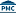Free Full Text.↩︎

10. FDA, CDER. NDA 204-412. Clinical Pharmacology and Biopharmaceutics Review(s). Reference ID: 3244307. Online.↩︎

11. AAPS, FDA, FIP, HPB, AOAC. Analytical Methods Validation: Bioavailability, Bioequivalence and Pharma­co­ki­netic Studies. Arlington, VA. December 3–5, 1990.↩︎

12. Think about paracetamol/acetaminophen. With its molecular mass 151.165 g·mol–1 and $$\small{f}$$ 0.75 after a 500 mg oral dose we start with ~4.94·1021 molecules in the circulation. Given its half life of 2½ hours after one week still ≈35 molecules happily float around.↩︎

13. The declared content $$\small{\neq}$$ the measured one. Furthermore, we can never be sure that the measured content is the true one. Don’t forget analytical (in)accuracy and (im)pre­cision. BTW, our method was validated for the test product and not for the reference. A dose-correction is only acceptable under certain conditions for the EMA.

14. Sheldon Lee Cooper likely is fine with –0.05129329. For most people 95% is easier to interpret.↩︎

15. McGilveray IJ, Dighe SV, French IW, Midha KK (eds). Bio­International ’89. Issues in the Evaluation of Bio­avail­ability Data. Toronto. October 1–4, 1989.↩︎

16. Keene ON. The log transformation is special. Stat Med. 1995; 14(8): 811–9. doi:10.1002/sim.4780140810.Open Access.↩︎

17. Mantel N. Do We Want Confidence Intervals Symmetrical About the Null Value? Bio­metrics. 1977; 33(4): 759–60.↩︎

18. Kirkwood TBL. Bioequivalence Testing — A Need to Rethink. Biometrics. 1981; 37(3): 589–91. doi:10.2307/2530573.↩︎

19. I can imagine what people would say:
»Of course, we do. It’s written in the holy books and the probability of passing increases – that’s great! If regulators don’t care about an inflated Type I Error, why should we?«↩︎

20. Schuirmann DJ. A comparison of the Two One-Sided Tests Procedure and the Power Approach for Assessing the Equivalence of Average Bioavailability. J Pharmacokin Bio­pharm. 1987; 15(6): 657–80. doi:10.1007/BF01068419.↩︎

21. Goldberg D. What Every Computer Scientist Should Know About Floating-Point Arithmetic. ACM Computing Surveys. 1991; 23(1): 5–48. doi:10.1145/103162.103163.Open Access.↩︎

22. Health Canada. Guidance Document. Comparative Bioavailability Standards: Formu­lations Used for Systemic Effects. Pub.:170501. Ottawa. 2018/06/08. Online.↩︎

23. MCC. Registration of Medicines. Biostudies. Pretoria. June 2015. Online.↩︎

24. GHC. The GCC Guidelines for Bioequivalence. DS-G-010-V03/110203. May 2021. Online.↩︎

25. League of Arab States, Higher Technical Committee for Arab Pharmaceutical Industry. Harmonised Arab Guideline on Bioequivalence of Generic Pharmaceutical Products. Cairo. March 2014. Online.↩︎

26. Karalis V, Symillides M, Macheras P. On the leveling-off properties of the new bioequivalence limits for highly variable drugs of the EMA guideline. Eur J Pharm Sci. 2011; 44: 497–505. doi:10.1016/j.ejps.2011.09.008.↩︎

27. Health Canada, Therapeutic Products Directorate. Notice: Policy on Bioequivalence Standards for Highly Vari­able Drug Products. File number 16-104293-140. Ottawa. April 18, 2016. Online.↩︎

28. FDA, OGD. Draft Guidance on Progesterone. Recommended Apr 2010, Revised Feb 2011. Download.↩︎

29. FDA, CDER. Draft Guidance for Industry. Bioequivalence Studies with Pharmacokinetic Endpoints for Drugs Sub­mitted Under an ANDA. Rockville. August 2021. Download.↩︎

30. McGilveray IJ, Midha KK, Skelly J, Dighe S, Doluisio JT, French IW, Karim A, Burford R. Consensus Report from “Bio International ’89”: Issues in the Evaluation of Bioavail­ability Data. J Pharm Sci. 1990, 79(10): 945–6. doi:10.1002/jps.2600791022.↩︎

31. Blume HH, Midha KK. Conference Report. Bio-International 92. Conference on Bio­avail­ability, Bioequivalence and Pharmacokinetic Studies. Bad Homburg, Germany, May 20–22, 1992. In: Midha KK, Blume HH (eds). Bio-Inter­na­tio­nal. Bioavailability, Bioequivalence and Pharmacokinetics. Stuttgart: medpharm; 1993.↩︎